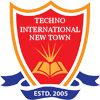#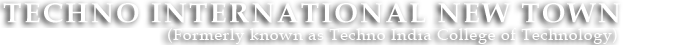+91 9674112076/2079
•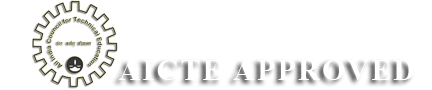•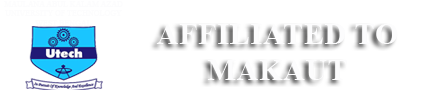•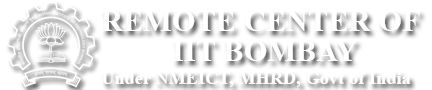•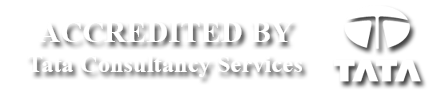# Digital Electronics Lab

ANALOG & DIGITAL ELECTRONIC CIRCUIT LABORATORY

Code: EC492

List of Experiments:

1. Realization of basic gates using Universal logic gates.
2. Code conversion circuits- BCD to Excess-3 and vice-versa.
3 Four-bit parity generator and comparator circuits.
4. Construction of simple Decoder and Multiplexer circuits using logic gates.
5. Design of combinational circuit for BCD to decimal conversion to drive 7-segment display using multiplexer.
6. Construction of simple arithmetic circuits-Adder, Subtractor.
7. Realization of RS-JK and D flip-flops using Universal logic gates.
8. Realization of Universal Register using JK flip-flops and logic gates.
9. Realization of Universal Register using multiplexer and flip-flops.
10. Realization of Asynchronous Up/Down counter.
11. Realization of Synchronous Up/Down counter.
12. Realization of Ring counter and Johnson’s counter.
14. Code conversion circuits – Binary to Gray & Vice-Versa. (Innovative)
15. Design of Sequential Counter with irregular sequences. (Innovative)

Code :EC (EE) 391

List of Experiments:

1. Study of Ripple and Regulation characteristics of full wave rectifier with and without capacitor filter.
2. Study of Zener diode as voltage regulator.
3. Construction of two stage R-C coupled amplifier & study of its gain and Bandwidth.
4. Study of class A, C & Push pull amplifier.
5. Realization V-I & I-V converter using Operational Amplifier.
6. Study of timer circuit using NE 555 and configuration of Mono-stable and Astable Multivibrator.
7. Study of DAC & ADC
8. Realization of basic gates using Universal logic gates.
9. Realization of RS-JK & D filp-flop using logic gates.
10. Design of Combinational circuit for BCD to decimal conversion to drive 7-segment display using Multiplexer.
11. Realization of Synchronous Up/Down counter.
12. Construction of simple Decoder & Multiplexer circuits using logic gates.